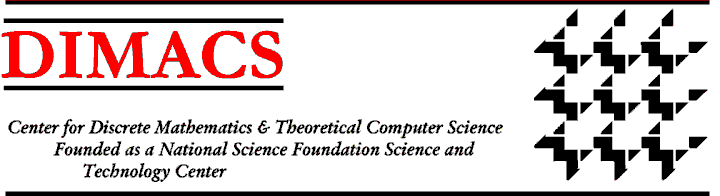### DIMACS Workshop on Discrete Mathematical Chemistry

#### March 23-25, 1998 Rutgers University

Organizers:
Pierre Hansen pierreh@crt.umontreal.ca
Patrick W. Fowler
Maolin Zheng
Discrete Mathematics have been used in chemistry for well over a century. Graph theory is a tool of choice to represent molecules (the name graph appears to have been coined by A. Cayley in the context of such studies), clusters or reaction paths. Group theory is much used for the study of molecular symmetries. Coding theory is basic in systematizing the enormous amount of chemical data available, in problems of enumeration of molecules, isomers, and families having various properties. Graph invariants are much used in Structure-Activity and Structure-Property Relationship studies. All these techniques are increasingly used by industry in the rapidly expanding fields of Computer-Assisted Molecular Design and Combinatorial Chemistry.

While there have long been contacts between chemists and mathematicians or computer scientists (a few of which do most of their research on problems from chemistry) much mathematical work is done independently by the chemists. This had led to new chemical theories but also to new concepts (e.g. the Randi\'c number in graph theory) and new algorithmic ideas. However, there has been, and still is, some duplication of effort.

The aim of the workshop would be to bring together

(i) chemists working in the mathematical chemistry field;
(ii) mathematicians and computer scientists working in that field;
(iii) mathematicians and computer scientists who do not yet work on chemical problems but whose research topic and/or recent results hold much potential for solving problems in chemistry,
(iv) chemists, mathematicians and computer scientists working in the chemical industry,

to discuss

(a) theoretical problems of chemistry expressed by discrete mathematics;
(b) chemical graph algorithms;
(c) coding theory applied to chemistry;
(d) applications of discrete mathematics in the chemical industry;
(e) open problems and directions for future research in discrete mathematical chemistry;
(f) software for discrete mathematical chemistry (with demonstrations).Next: Call for ParticipationWorkshop IndexDIMACS Homepage
Contacting the Center